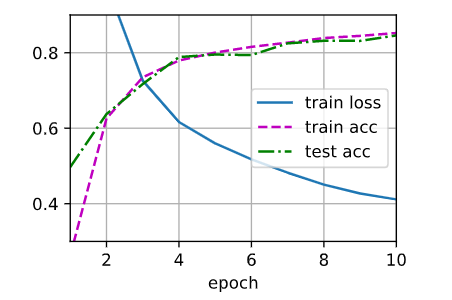# 多层感知机的从零实现

torch.max(input, other, out=None) → Tensor

input (Tensor) – 输入张量
other (Tensor) – 输出张量
out (Tensor, optional) – 结果张量

a = torch.randn(4)
a

1.3869
0.3912
-0.8634
-0.5468
[torch.FloatTensor of size 4]

b = torch.randn(4)
b

1.0067
-0.8010
0.6258
0.3627
[torch.FloatTensor of size 4]

torch.max(a, b)

1.3869
0.3912
0.6258
0.3627
[torch.FloatTensor of size 4]

RuntimeError: DataLoader worker (pid(s) 20584, 44860) exited unexpectedly

test_iter.num_workers = 0
train_iter.num_workers = 0

Original: one hidden, num=256, epoch=10, lr=0.1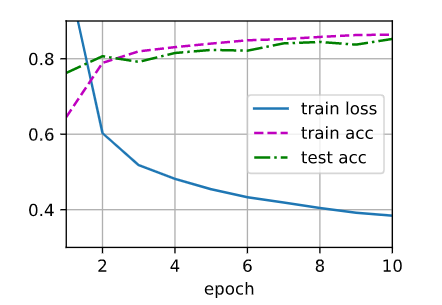Test1) Change num_hiddens=512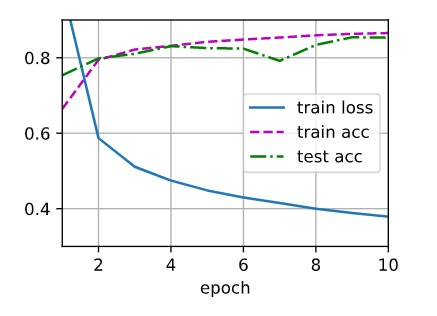Test2) Change epoch=20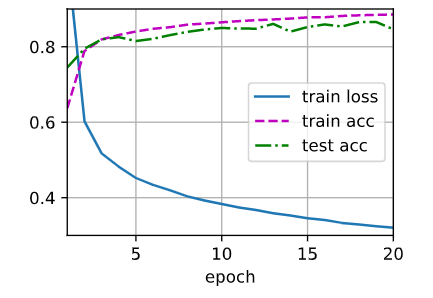Test3) Change lr=0.05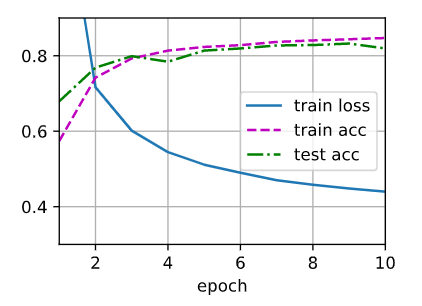Test4) Adding second hidden layer num=64